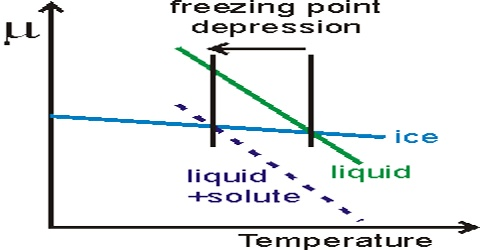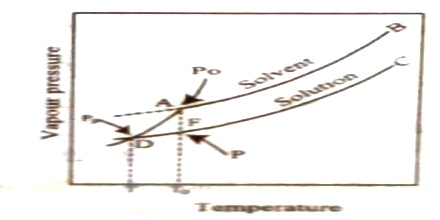# Depression of Freezing Point

Depression of Freezing Point

The freezing point of a liquid is defined as the temperature at which the liquid and solid forms are in equilibrium and the freezing point of a solution is the temperature at which the solution is in equilibrium with the pure crystalline solvent. The depression of freezing point of a liquid by the addition of a non-volatile and non-electrolytic solute may be discussed more conveniently with the help of vapour pressure-temperature curves as in Figure. The vapour pressure curves of liquid solvent and solid solvent meet at A. Hence this corresponds to the freezing point T0 of the solvent. D corresponds to the freezing point T of the solution as this is the temperature at which the solution and solid solvent have the same vapour pressure. It is clear that T is lower than T0 and the freezing points of solutions will always be lower than those of pure solvent as the vapour pressure curves for the former lie below that for the latter.Fig: Depression of Freezing Point

A quantitative relationship between the concentration of solute in solution and the depression of freezing point was given by F.M. Raoult (1878-86) to the form of two laws which are generally known as Raoult’s laws of depression of freezing point.

(i) The depression of freezing point of a solvent due to the addition of a solute is proportional to the molality, m, of the solution. Expressed mathematically,

∆Tf  = Kf.m

where Kf is a constant known as the molal depression of freezing Point constant or cryoscopic constant.

(ii) Equimolecular quantities of different substance, dissolved in the same quantity of the same solvent lower the freezing point to the same extent.

It may be noted that these laws are similar to the laws of boiling point elevation and have the same limitations as those, i.e., these are applicable to dilute solutions of non-volatile non-electrolytes, which do not undergo association or dissociation in solution. An additional condition is that during freezing only pure solvent should separate as the solid phase.